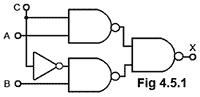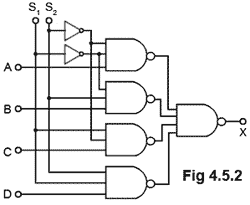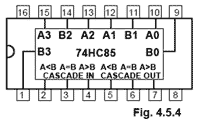# Combinational Logic Quiz

Try our quiz, based on the information you can find in Digital Electronics Module 4 - Combinational Logic. Submit your answers and see how many you get right. If you get any answers wrong, just follow the hints to find the right answer and learn about the combinational logic circuits as you go.

### 1.

If the inputs to a full adder are A=1, B=0, CIN=1 what will be the logic states on the outputs S and COUT?

### 2.

In a two-gate half adder circuit, which of the listed gates would produce a Carry output?

### 3.What type of circuit is illustrated in to Fig 4.5.1?

### 4.Refer to Fig. 4.5.2, If S1=1 and S2= 0 what will be the logic state at the output X?

### 5.Refer to Fig 4.5.3. If A = 0 and B = 1, what will be the logic states at X, Y and Z?

### 6.What is the integration scale of the IC illustrated in Fig 4.5.4?

### 7.

Refer to Fig 4.5.4. When two or more ICs of the type illustrated are connected in cascade, what should be the logic states applied to pins 2, 3 and 4 of the IC processing the least significant 4 bits?

### 8.What type of combinational logic circuit is illustrated in Fig. 4.4.5?

### 9.

What is the main purpose of the Ripple Blanking facility on some decoder ICs?

### 10.

What is the range of memory that can be addressed in a memory system, using a 16-bit address bus, 8Kbyte memory chips and a single 3 to 8 line address decoder?

Top of Page